## Introduction

• A prism is a three-dimensional solid (polyhedron) with a pair of identical and parallel faces at opposite ends (called bases of the prism).
• A prism is named after its base.
• There are different prisms named based on the shape of their bases. Here are some examples: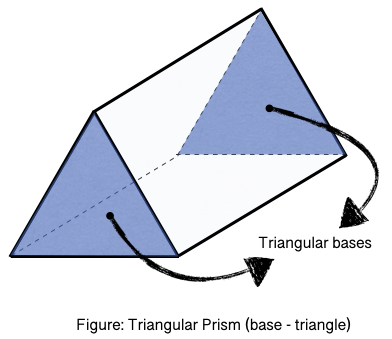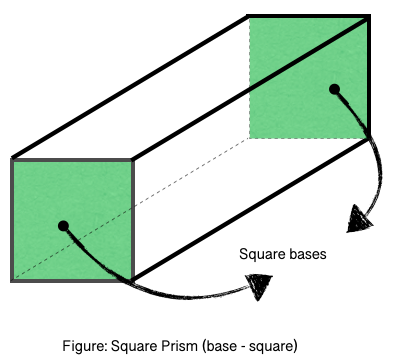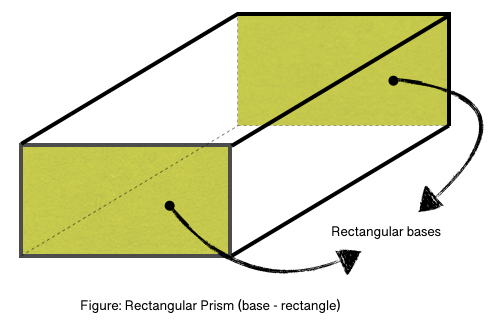• The height $\left(h\right)$ of a prism is the perpendicular distance between the two bases.

## Volume of a Triangular Prism

• A prism's volume with the area of the base $\left(B\right)$ and height $\left(h\right)$ can be calculated using the formula mentioned below.

$Volume=B×h$

• For a triangular prism with a base having altitude $\left(a\right)$, length of the base $\left(b\right)$, and height $\left(h\right)$: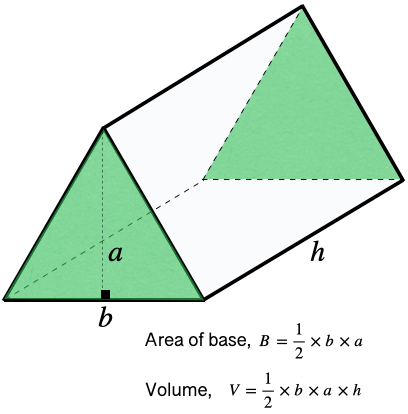• For a triangular prism with a base having equal sides $\left(s\right)$: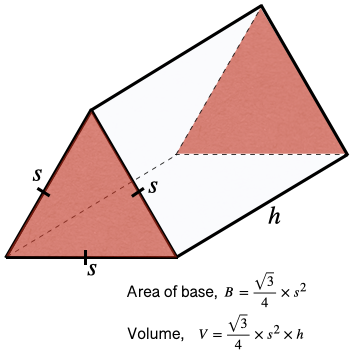## Surface Area of a Triangular Prism

• A triangular prism has 2 triangular bases and 3 lateral faces (parallelogram).
• The lateral area of a triangular prism: $LA=p×h$, where $p=$the perimeter of the base
• The total area of a triangular prism: $TA=LA+2B$$=p×h+2B$

## Volume of a Rectangular/Square Prism

• A prism's volume with the area of the base $\left(B\right)$ and height $\left(h\right)$ can be calculated using the formula mentioned below.

$Volume=B×h$

• For a rectangular prism (cuboid) with a base having length $\left(l\right)$, width $\left(w\right)$, and height $\left(h\right)$: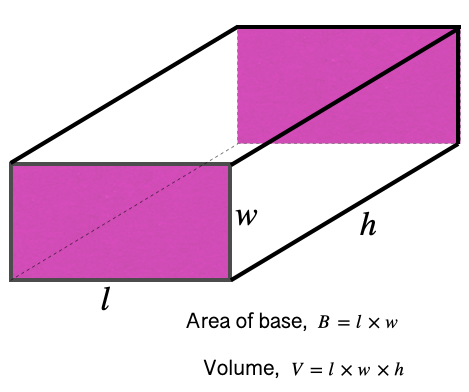• For a square prism (cuboid) with a base having equal sides $\left(s\right)$: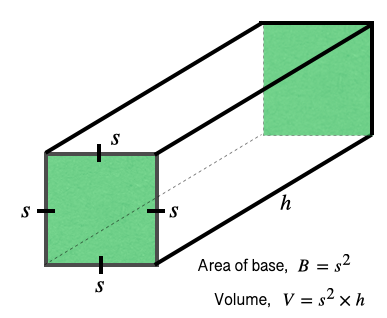## Surface Area of a Rectangular/Square Prism

• A rectangular prism has 2 rectangular bases and 4 lateral faces (parallelogram).
• A square prism has 2 square bases and 4 lateral faces (parallelogram).
• The lateral area of a rectangular/square prism: $LA=p×h$, where $p=$the perimeter of the base
• The total area of a rectangular/square prism: $TA=LA+2B$$=p×h+2B$

## Solved Examples

Question 1: Find the volume of the following triangular prism.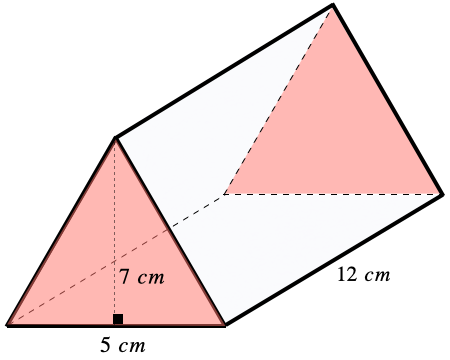Solution: $Volum{e}_{prism}=B×h$

$=\frac{1}{2}×b×a×h$

$=\frac{1}{2}×5×7×12$

$=5×7×6$

Question 2: Find the total surface area of the rectangular prism shown below.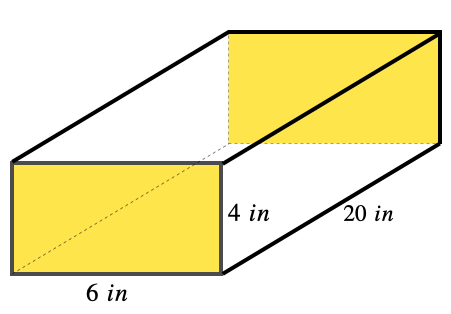Solution: $T{A}_{prism}=p×h+2B$

$=2\left(6+4\right)×20+2\left(6×4\right)$

$=20×20+2×10$

$=400+20$

## Cheat Sheet

• For any prism with the area of base $\left(B\right)$, height $\left(h\right)$, and perimeter of base $\left(p\right)$:
• Volume, $Volum{e}_{prism}=B×h$
• Lateral Surface Area, $L{A}_{prism}=p×h$
• Total Surface Area, $T{A}_{prism}=L{A}_{prism}+2B$$=p×h+2B$

## Blunder Areas

• In finding the volume or surface area of a triangular prism, students need to differentiate between the triangle's height (altitude) and the prism's height.
• A prism can have triangle, square, rectangular, pentagonal, and other polygon shapes as the base but not curved ones such as a circle.